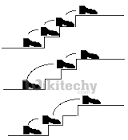# C Programming – Count ways to reach the n’th stair

C Programming - Count ways to reach the n’th stair - Dynamic Programming There are n stairs, a person standing at the bottom wants to reach the top.

There are n stairs, a person standing at the bottom wants to reach the top. The person can climb either 1 stair or 2 stairs at a time. Count the number of ways, the person can reach the top.Consider the example shown in diagram. The value of n is 3. There are 3 ways to reach the top. The diagram is taken from Easier Fibonacci puzzles

More Examples:

```Input: n = 1
Output: 1
There is only one way to climb 1 stair

Input: n = 2
Output: 2
There are two ways: (1, 1) and (2)

Input: n = 4
Output: 5
(1, 1, 1, 1), (1, 1, 2), (2, 1, 1), (1, 2, 1), (2, 2)```

We can easily find recursive nature in above problem. The person can reach n’th stair from either (n-1)’th stair or from (n-2)’th stair. Let the total number of ways to reach n’t stair be ‘ways(n)’. The value of ‘ways(n)’ can be written as following.

```    ways(n) = ways(n-1) + ways(n-2)
```

The above expression is actually the expression for Fibonacci numbers, but there is one thing to notice, the value of ways(n) is equal to fibonacci(n+1).

ways(1) = fib(2) = 1
ways(2) = fib(3) = 2
ways(3) = fib(4) = 3

So we can use function for fibonacci numbers to find the value of ways(n). Following is C++ implementation of the above idea.

C
``````// A C program to count number of ways to reach n't stair when
// a person can climb 1, 2, ..m stairs at a time.
#include<stdio.h>

// A simple recursive program to find n'th fibonacci number
int fib(int n)
{
if (n <= 1)
return n;
return fib(n-1) + fib(n-2);
}

// Returns number of ways to reach s'th stair
int countWays(int s)
{
return fib(s + 1);
}

// Driver program to test above functions
int main ()
{
int s = 4;
printf("Number of ways = %d", countWays(s));
getchar();
return 0;
}``````

Output:

`Number of ways = 5`

The time complexity of the above implementation is exponential (golden ratio raised to power n). It can be optimized to work in O(Logn) time using the previously discussed Fibonacci function optimizations.

Generalization of the above problem
How to count number of ways if the person can climb up to m stairs for a given value m? For example if m is 4, the person can climb 1 stair or 2 stairs or 3 stairs or 4 stairs at a time.

We can write the recurrence as following.

`   ways(n, m) = ways(n-1, m) + ways(n-2, m) + ... ways(n-m, m)`

Following is C++ implementation of above recurrence.

C
``````// A C program to count number of ways to reach n't stair when
// a person can climb either 1 or 2 stairs at a time
#include<stdio.h>

// A recursive function used by countWays
int countWaysUtil(int n, int m)
{
if (n <= 1)
return n;
int res = 0;
for (int i = 1; i<=m && i<=n; i++)
res += countWaysUtil(n-i, m);
return res;
}

// Returns number of ways to reach s'th stair
int countWays(int s, int m)
{
return countWaysUtil(s+1, m);
}

// Driver program to test above functions
int main ()
{
int s = 4, m = 2;
printf("Nuber of ways = %d", countWays(s, m));
return 0;
}``````

Output:

`Number of ways = 5`

The time complexity of above solution is exponential. It can be optimized to O(mn) by using dynamic programming. Following is dynamic programming based solution. We build a table res[] in bottom up manner.

C
``````// A C program to count number of ways to reach n't stair when
// a person can climb 1, 2, ..m stairs at a time
#include<stdio.h>

// A recursive function used by countWays
int countWaysUtil(int n, int m)
{
int res[n];
res = 1; res = 1;
for (int i=2; i<n; i++)
{
res[i] = 0;
for (int j=1; j<=m && j<=i; j++)
res[i] += res[i-j];
}
return res[n-1];
}

// Returns number of ways to reach s'th stair
int countWays(int s, int m)
{
return countWaysUtil(s+1, m);
}

// Driver program to test above functions
int main ()
{
int s = 4, m = 2;
printf("Nuber of ways = %d", countWays(s, m));
return 0;
}``````

Output:

`Number of ways = 5`